# How to Create a Venn Diagram in R (8 Examples)

This article illustrates how to draw venn diagrams in the R programming language. Venn diagrams are also referred to as primary diagram, set diagram, or logic diagram.

Let’s do this:

## Setting Up VennDiagram Package

In the examples of this R tutorial, we’ll use functions provided by the VennDiagram add-on package for the R programming language. In order to use the functions of VennDiagram, we need to install and load the package first:

```install.packages("VennDiagram")                       # Install VennDiagram package

I recommend to have a look at the help documentation of the VennDiagram package. However, in the following examples you’ll learn how to apply the main functions of the VennDiagram package.

## Example 1: Single Venn Diagram in R

Example 1 shows how to draw a single venn diagram, i.e. only one circle. Have a look at the following R code:

```grid.newpage()                                        # Move to new plotting page
draw.single.venn(area = 10)                           # Create single venn diagram```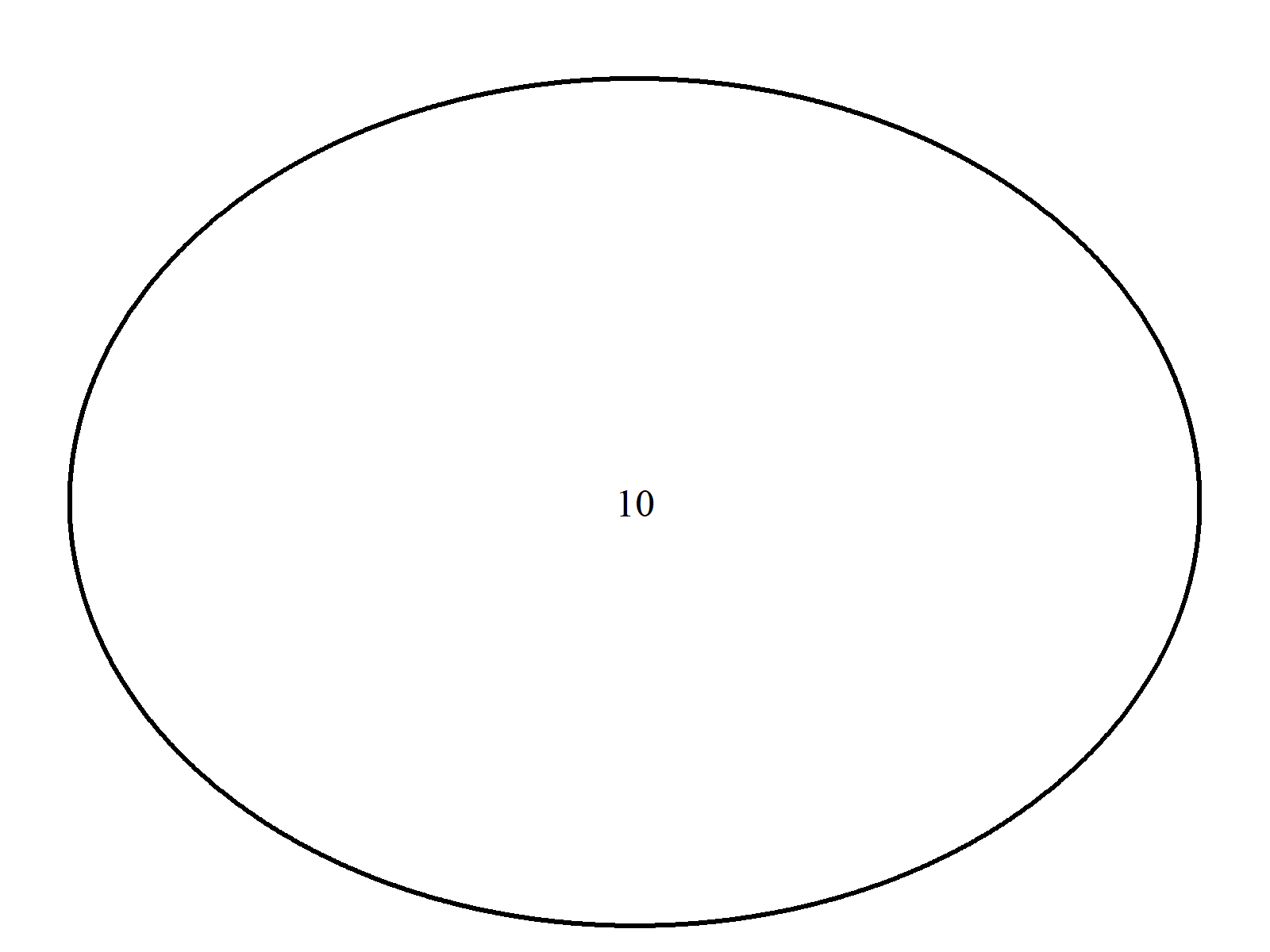Figure 1: Single Venn Diagram in R.

Figure 1 is visualizing the output of the previous R syntax. Note that the previous code contains two steps.

First, we are creating a new plotting page with the grid.newpage function. We should usually do this step before the creation of each venn diagram, because otherwise the venn diagram is just overlaying previously created plots.

Second, we are producing our single venn diagram with the draw.single.venn function. All we are specifying within the function is the size of our area (i.e. 10).

## Example 2: Pairwise Venn Diagram

The VennDiagram package provides functions for the production of venn diagrams of basically every number of sets (i.e. circles). The draw.pairwise.venn is used to draw pairwise venn diagrams.

If we want to apply the draw.pairwise.venn command, we need to specify the sizes of the areas of both sets as well as the intersection of the two sets:

```grid.newpage()                                        # Move to new plotting page
draw.pairwise.venn(area1 = 10,                        # Create pairwise venn diagram
area2 = 20,
cross.area = 2)```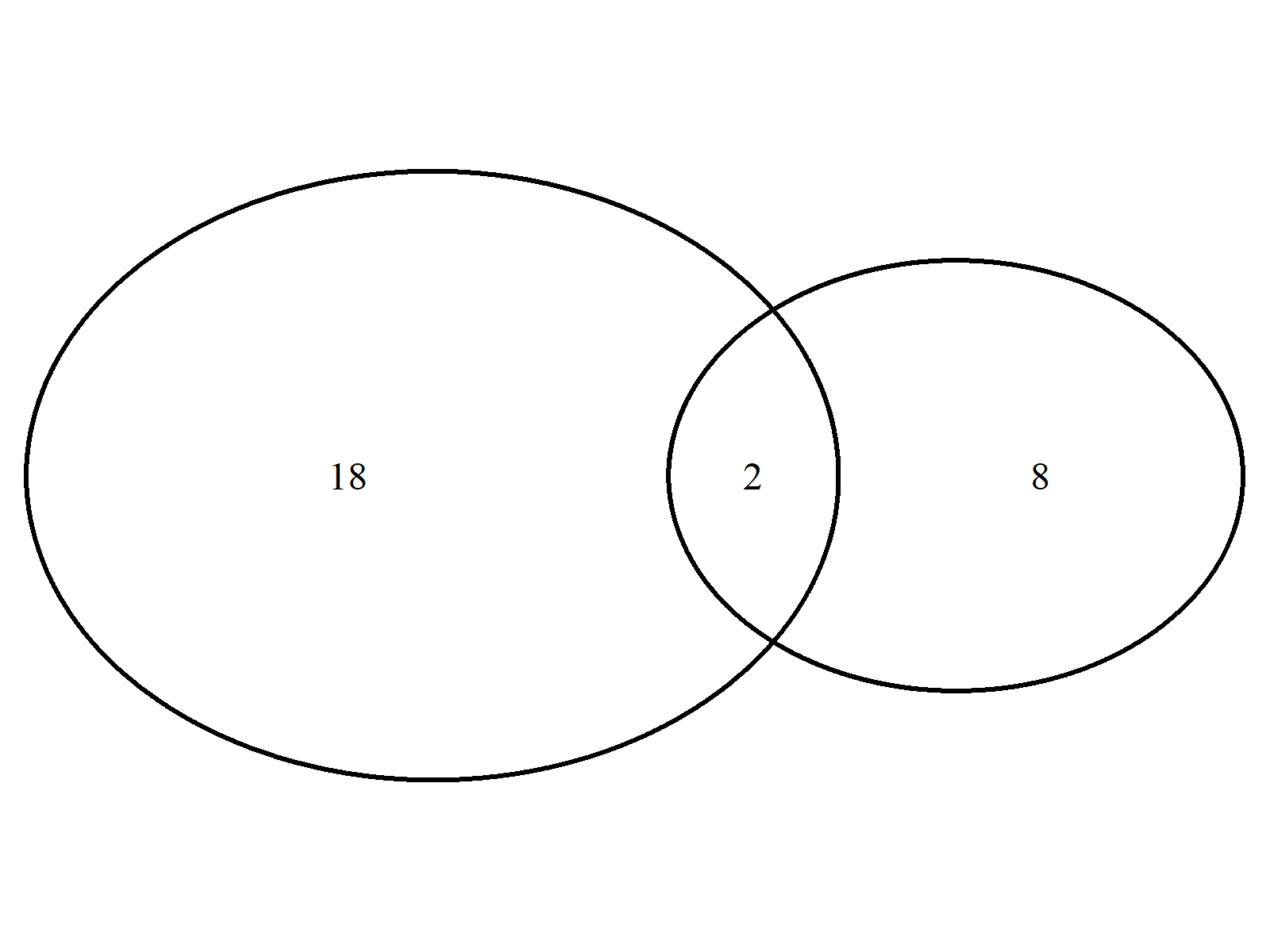Figure 2: Pairwise Venn Diagram.

Figure 2 is showing the output of our previous R code. As you can see, the size of the areas are reflected in the visualization of the pairwise venn diagram.

## Example 3: Venn Diagram with Three Sets

Similar to the R programming code of Example 2, we can use the draw.triple.venn function to create a venn diagram with three sets. Note that this time we need to specify three different area values as well as the pairwise intersections and the intersection area of all sets:

```grid.newpage()                                        # Move to new plotting page
draw.triple.venn(area1 = 10,                          # Create venn diagram with three sets
area2 = 20,
area3 = 15,
n12 = 2,
n23 = 3,
n13 = 7,
n123 = 2)```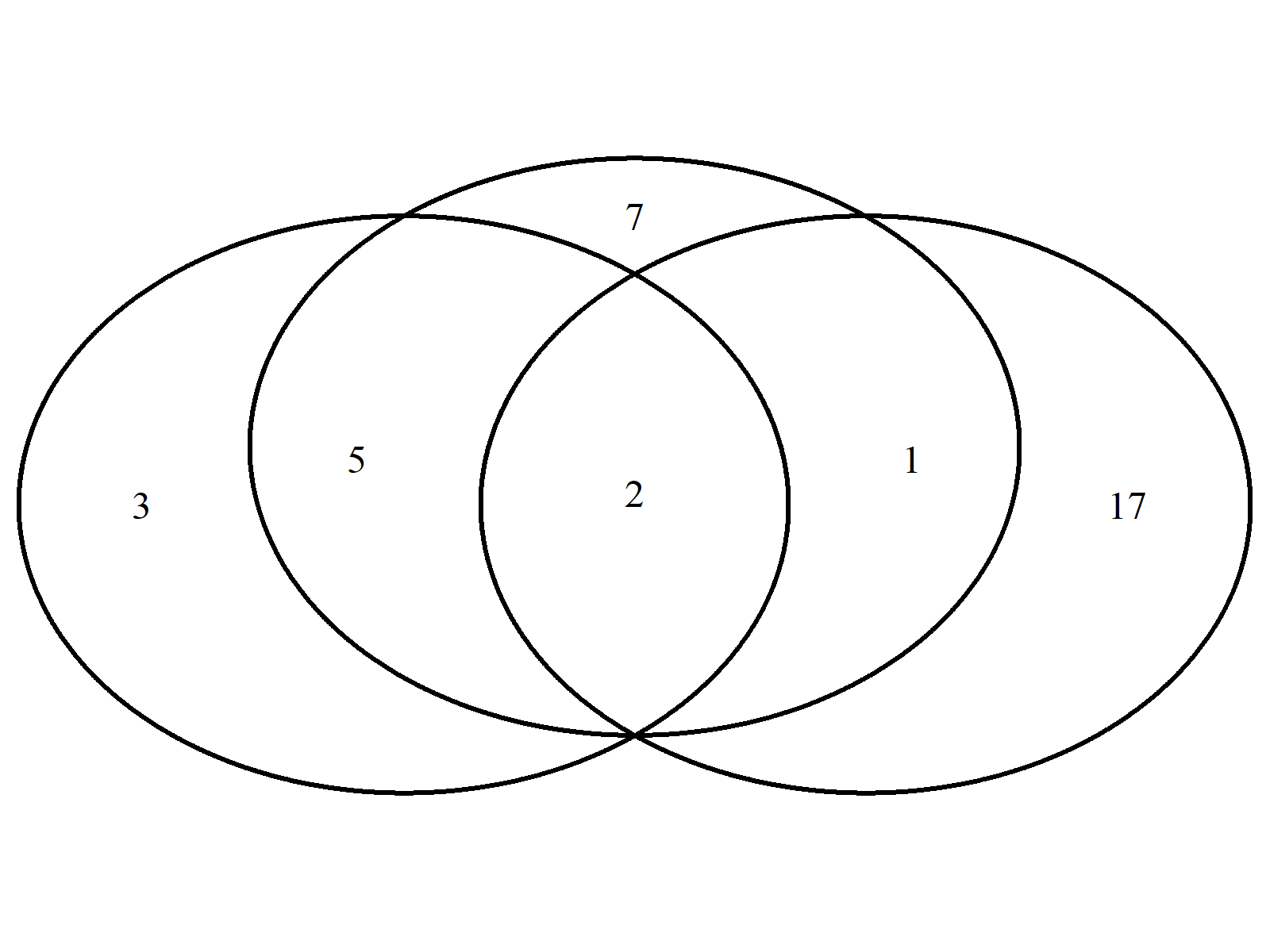Figure 3: Triple Venn Diagram.

Note that the VennDiagram package provides further functions for more complex venn diagrams with multiple sets, i.e. draw.quad.venn, draw.quintuple.venn, or the more general function venn.diagram, which is taking a list and creates a TIFF-file in publication-quality.

However, for simplicity we’ll stick to the triple venn diagram in the remaining examples of this R tutorial.

## Example 4: Change Color of Venn Diagram

In Example 4, I’ll show you how to make a venn diagram with colored lines around the circles and a filling color of the circles. The following R code is the same as in Example 3, but in addition we are specifying the line color to be red and the filling color to be blue (with the HEX-code #1b98e0). Have a look at the output:

```grid.newpage()                                        # Move to new plotting page
draw.triple.venn(area1 = 10,                          # Change color of venn diagram
area2 = 20,
area3 = 15,
n12 = 2,
n23 = 3,
n13 = 7,
n123 = 2,
col = "red",
fill = "#1b98e0")```Figure 4: Venn Diagram with Color.

## Example 5: Specify Different Color for Each Set

We can also specify a different color for each of the sets of our venn diagram. For this task, we need to set the fill argument to be equal to a vector of colors. Each element of this vector is defining the color of one of the circles:

```grid.newpage()                                        # Move to new plotting page
draw.triple.venn(area1 = 10,                          # Different color for each set
area2 = 20,
area3 = 15,
n12 = 2,
n23 = 3,
n13 = 7,
n123 = 2,
col = "red",
fill = c("pink", "green", "orange"))```Figure 5: Different Color for Each Circle.

## Example 6: Disable Transparency of Venn Diagram

You may have noticed that the previous venn diagrams are transparent, i.e. showing the intersections in a mixed and overlapping color. If we want to reduce or even disable this transparency, we can use the alpha argument of the VennDiagram functions. An alpha of 1, as shown below, is disabling the transparency completely:

```grid.newpage()                                        # Move to new plotting page
draw.triple.venn(area1 = 10,                          # Disable transparency of venn diagram
area2 = 20,
area3 = 15,
n12 = 2,
n23 = 3,
n13 = 7,
n123 = 2,
col = "red",
fill = c("pink", "green", "orange"),
alpha = 1)```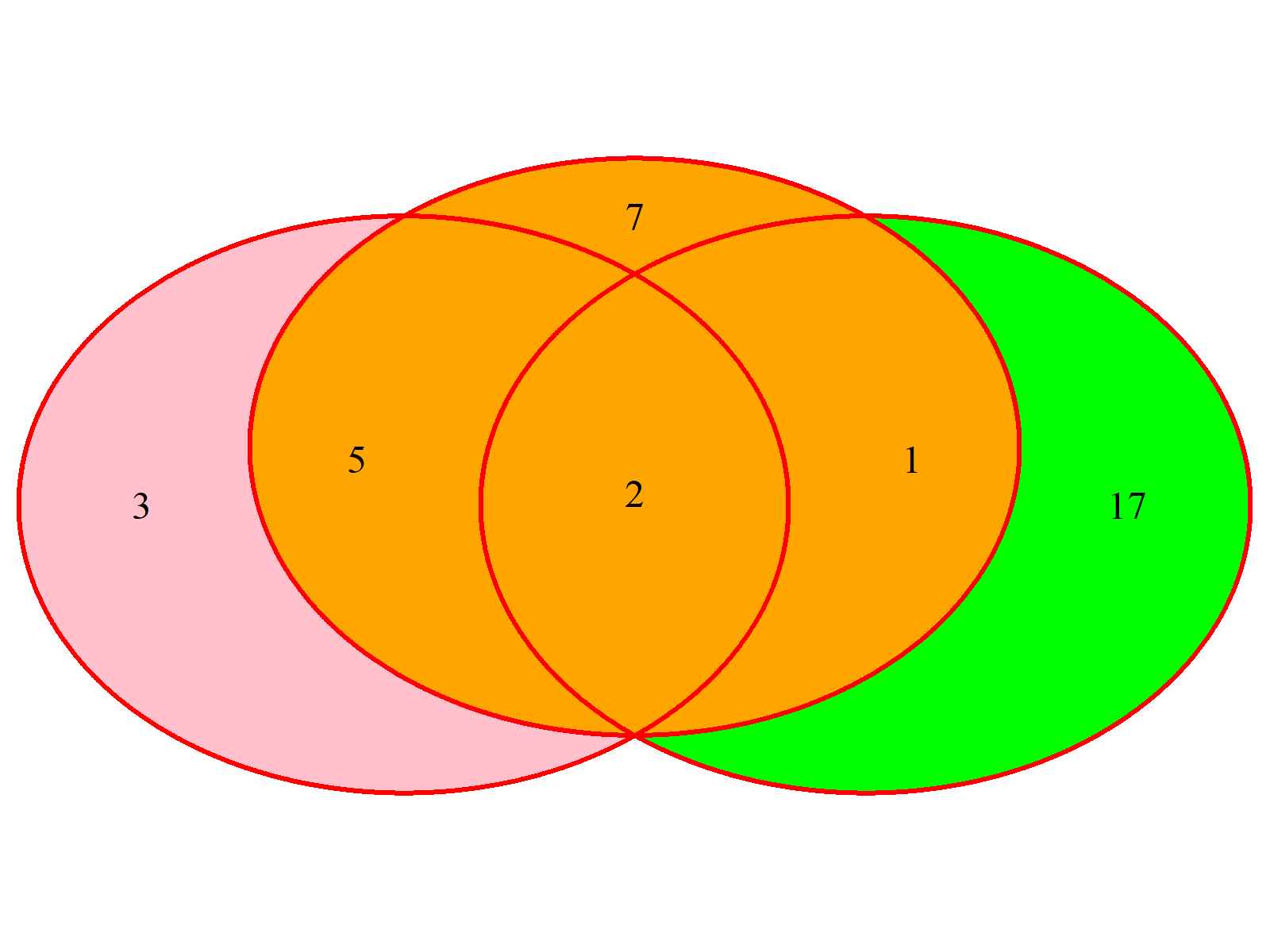Figure 6: No Transparency in Venn Diagram.

## Example 7: Remove Lines from Venn Diagram

The VennDiagram functions provide the possibility to remove the lines around the circles by specifying the lty argument to be equal to blank. Let’s do this in practice:

```grid.newpage()                                        # Move to new plotting page
draw.triple.venn(area1 = 10,                          # Remove lines from venn diagram
area2 = 20,
area3 = 15,
n12 = 2,
n23 = 3,
n13 = 7,
n123 = 2,
fill = c("pink", "green", "orange"),
lty = "blank")```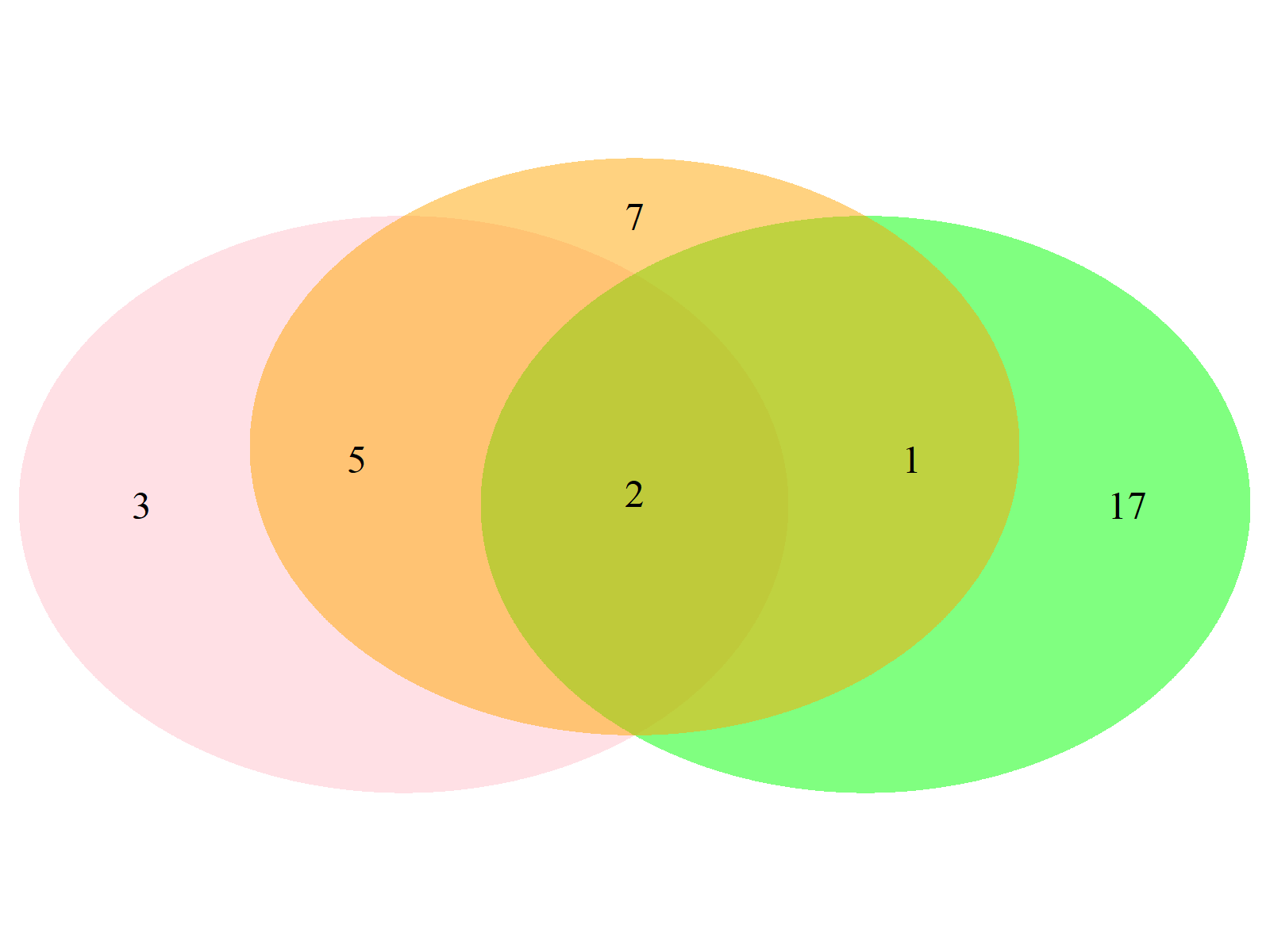Figure 7: Venn Diagram without Lines.

## Example 8: Add Name to Each Set of Venn Diagram

Finally, I want to show you how to assign category names (or labels) to each of our sets. We can do that by assigning a vector of category names to the category option of the VennDiagram functions:

```grid.newpage()                                        # Move to new plotting page
draw.triple.venn(area1 = 10,                          # Add name to each set
area2 = 20,
area3 = 15,
n12 = 2,
n23 = 3,
n13 = 7,
n123 = 2,
fill = c("pink", "green", "orange"),
lty = "blank",
category = c("Group 1", "Group 2", "Group 3"))```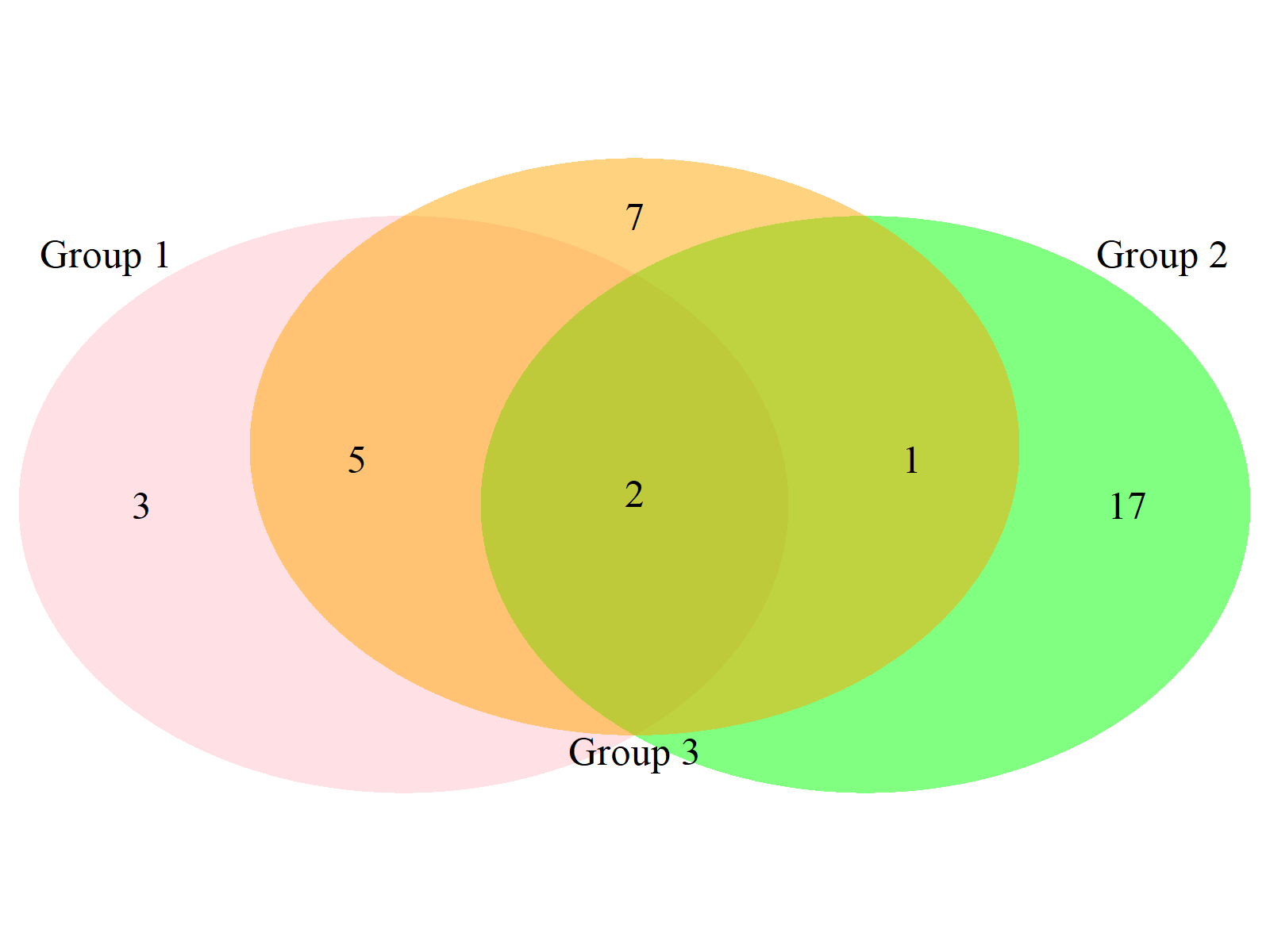Figure 8 is the same as Figure 7, but this time it is showing labels for each circle.

## Video, Further Resources & Summary

If you need further info on the examples of this page, you might want to watch the following video which I have published on my YouTube channel. In the video, I illustrate the R codes of this tutorial.

Please accept YouTube cookies to play this video. By accepting you will be accessing content from YouTube, a service provided by an external third party.If you accept this notice, your choice will be saved and the page will refresh.

Subscribe to the Statistics Globe Newsletter

•Elisee
May 4, 2020 10:33 am

Hi Mr. As you know in Venn Diagramm we have to put some words in every venn. I would like to know how to do it with R

•May 4, 2020 2:07 pm

Hi Elisee,

That’s actually a bit tricky (as far as I know). I found this forum thread on Stack Overflow, based on which I created the following R code:

```grid.newpage()                                                       # Move to new plotting page
venn.plot <- draw.pairwise.venn(area1 = 10,                          # Create pairwise venn diagram
area2 = 20,
cross.area = 2)

addlab <- function(lab, x, y, offset = 0) {                          # Create own function
grid.text(lab, unit(as.numeric(x), "npc"),
unit(as.numeric(y) - offset, "npc"),
draw = FALSE)
}

my_labels <- c("text_1", "text_2", "text_3", "text_4", "text_5")     # Define text for each element
lbls <- gList()
text_counter <- 1

for(i in seq(along.with = venn.plot)) {
if(regexpr("text", venn.plot[[i]]\$name) > 0) {                     # Check if it is a grid.text object
lbls <- gList(lbls,                                              # Write counter value below existing label
venn.plot[[i]]\$x,
venn.plot[[i]]\$y,
0.03))
text_counter <- text_counter + 1                                 # Increase counter
}
}

grid.draw(venn.plot)                                                 # Draw venn diagram

You may replace text_1… by any text you want.

I hope that helps!

Joachim

•Jim Roper
June 15, 2020 9:11 pm

In the triple venn, the three ovals or circles are equal in size, rather than proportional to the dimensions that I put in. Is there a way to make them proportional?

•June 16, 2020 1:27 pm

Hi Jim,

Thanks for the question! Unfortunately, I don’t know by heart how to do this and a Google Search revealed that this is a bit tricky. I found this thread on Stack Overflow, which is discussing the topic.

I hope that helps!

Joachim

•Jim Roper
June 15, 2020 9:54 pm

Another thing, many journals want figures with sanserif fonts. Is there a way to change the font type of the numbers that are automatically displayed?

•June 16, 2020 1:30 pm

Hi again 🙂

I assume this should be possible by specifying the font options when exporting the figures (e.g. in the pdf() function). Have a look at this thread for more information.

Regards,

Joachim

•George Wasonga Alal
March 21, 2021 5:22 pm

Hello Joachim,
How do I arrange a .csv file to draw a Venn diagram with multiple variables i.e.
1. Classes of Bacteria in Tissues clone Libraries
2. Classes of Bacteria in Water Clone Libraries and
3. “Classes of bacteria shared between Tissue and water clone libraries”

•March 22, 2021 8:16 am

Hi George,

I would calculate the number of cases in each of your groups using logical conditions. For example:

`nrow(data[data\$class == "Tissues", ])`

The resulting number of cases would then be used as area values as explained in this tutorial.

I hope that helps!

Joachim

•George Wasonga Alal
March 23, 2021 2:52 pm

Hello Joachim
Thanks for the help but if I have a data with many Row number but in three categories as stated above, how would you go about it? and these are in percentages

I can send the data and you let me know how you go about it with stacking pie chats as per the example shown with many pie charts stacked on each other

•March 23, 2021 3:49 pm

Hi George,

You may send me a subsample of your data so that I can have a look. You can find my email contact on the about page.

Regards

Joachim

•Alvaro
May 12, 2021 9:42 pm

Hello. What is the code to have two or more Venn diagram images on the same page (similar to the par function)?

•Aline Ruiz
July 8, 2021 9:23 pm

I have a question. I’m trying to make a Venn digram with nine sets but with the package just can do with five sets. Do you know how to make a nine-set Venn plot in R?

•July 9, 2021 6:59 am

Hey Aline,

As far as I know the venn package can draw venn diagrams up to seven sets (see here: https://cran.r-project.org/web/packages/venn/venn.pdf)

Unfortunately, I don’t know how to draw even more sets. Please let me know in case you find a solution, I’d also be curious if this is possible.

Regards

Joachim

•Amir
August 18, 2021 8:49 pm

Hello Joachim,
Thanks for these nice tutorials! I need to make a Venn diagram with 26 different combinations (20 intersections) which is derived from 6 different options, are there any options within venndiagram package to let me build such a figure?

Thanks

•August 19, 2021 2:22 pm

Hey Amir,

Thanks a lot for the kind feedback!

The venn package can draw venn diagrams with up to seven sets, but I don’t know any packages that can draw even more sets.

In case you are interested in the venn package, you may have a look at this tutorial: https://statisticsglobe.com/venn-r-package

Regards

Joachim

•amir
September 7, 2021 2:14 pm

•September 7, 2021 2:15 pm

You are very welcome Amir! 🙂

•Belle
February 9, 2022 10:43 am

Hi, thanks for the tutorial. Have you got one like this on how to make a Venn with 4 and 5 sets please? 🙂

•February 10, 2022 6:41 am

Hey Belle,

Thank you, glad it is useful to you! 🙂

The venn package provides venn diagrams up to seven sets. You may find more info here.

Regards,
Joachim

•David Asare Kumi
February 18, 2022 12:08 am

I would like to know if you can partition one set into three, say A, B, and C and in each you have a D as in the case of computing Bayes Theorem.
Once again, I think you so very much for your good work.

•February 18, 2022 9:08 am

Hey David,

Thank you very much for this wonderful feedback, glad you like my tutorials! 🙂

Could you share an image that looks like the one you want to create?

Regards,
Joachim

•Paria
May 20, 2022 1:42 pm

Dear Joachim Schork,
Thank you for your step-by-step instruction.
I am drawing a Venn diagram for three different sets of gene names, with VennDiagram Package.
Do you know how I can get the lists of the overlapping gene names?

•May 20, 2022 2:50 pm

Hey Paria,

Thanks for the kind words, glad you like the tutorial!

I think the easiest way to do this is to check those overlaps directly in your data set, and not based on the Venn Diagram.

Could you share the structure of your data? What is returned when you run the following code?

`head(your_data)`

Regards,
Joachim

•Puja
June 9, 2022 4:40 pm

Hi Joachim,

I am using the VennDiagram package and the diagram is fine. But I don’t know why the total numbers are not matching from the original datasets. Suppose, A=762 & B=641 \$ overlapping is 222, then the unique numbers in A & B circles should be 540 and 419 respectively. But I am not sure why the unique circle in B gives 420, adding 1 extra. Please help me in resolving this issue. Thanks.

•June 10, 2022 7:14 am

Hey Puja,

Regards,
Joachim

•John Perry
February 26, 2023 7:13 pm

How do you deal with (A U B U C)’ i.e. A union B union C complement???/

•February 27, 2023 9:26 am

Hello John,

Unfortunately, I am not familiar with this topic. However, on the web, I found this thread on StackOverflow. Maybe it helps.

Regards,
Cansu

•Tena
May 17, 2023 2:11 am

I am struggling with R. So, can you help me get the right script? If I have an Excel file has five columns for females ( Female 1, Female 2, Female 3, Female 4, Female 5) and five columns for males (Male 1, Male 2, Male 3, Male 4, Male 5), can I get vann diagram from this data?

•May 19, 2023 10:14 am

Hello Tena,

What do you have in each column?

Regards,
Cansu

•Tena
May 19, 2023 11:36 pm

Thank you very much for your help.
It is proteomic data for ten samples; we have more than 2000 proteins. So the amount of all protein for each sample is in each column. Also, there are common between males and females, and others are unique for each gender.

•May 22, 2023 7:44 am

Hello Tena,

So each column represents a sample, and they are named Female1, Female2, etc. What do you want to show in your visualization? I am asking because when it comes to more than four groups, which is 10 in your case, things get complicated with Venn diagrams in R. Maybe we can use another visual that serves your purpose.

Regards,
Cansu

•Tena
May 22, 2023 3:29 pm

I want to get the common between males and females and the unique quantity of proteins for males and females. If there is another option, please let me know about it. Have a great day

•May 23, 2023 8:31 am

Hello Tena,

Alternatively, you can use stacked barplots or heatmaps. I will give you the barplot example. Please be aware that I labeled each count per gender per protein to obtain a visual comparable with Venn diagrams.

```# install.packages("tidyverse")  # Uncomment to install the package if you haven't already
library(tidyverse)

# Sample data
set.seed(123) # for reproducibility

Female1 <- sample(LETTERS[1:5], 20, replace = TRUE)
Female2 <- sample(LETTERS[2:6], 20, replace = TRUE)
Female3 <- sample(LETTERS[1:6], 20, replace = TRUE)
Female4 <- sample(LETTERS[1:4], 20, replace = TRUE)
Female5 <- sample(LETTERS[3:7], 20, replace = TRUE)

Male1 <- sample(LETTERS[1:5], 20, replace = TRUE)
Male2 <- sample(LETTERS[1:4], 20, replace = TRUE)
Male3 <- sample(LETTERS[3:7], 20, replace = TRUE)
Male4 <- sample(LETTERS[2:6], 20, replace = TRUE)
Male5 <- sample(LETTERS[1:6], 20, replace = TRUE)

df <- data.frame(Female1, Female2, Female3, Female4, Female5, Male1, Male2, Male3, Male4, Male5)

# Convert to long format using tidyr's pivot_longer
df_long <- df %>%
pivot_longer(everything(), names_to = "Group", values_to = "Protein")

# Count occurrence of each protein in each group
df_count <- df_long %>%
count(Group, Protein)

# Create the plot
ggplot(df_count, aes(x = Group, y = n, fill = Protein)) +
geom_bar(stat = "identity") +
geom_text(aes(label = n), position = position_stack(vjust = 0.5)) +
theme(axis.text.x = element_text(angle = 45, hjust = 1)) +
labs(x = "Group", y = "Count", fill = "Protein type")```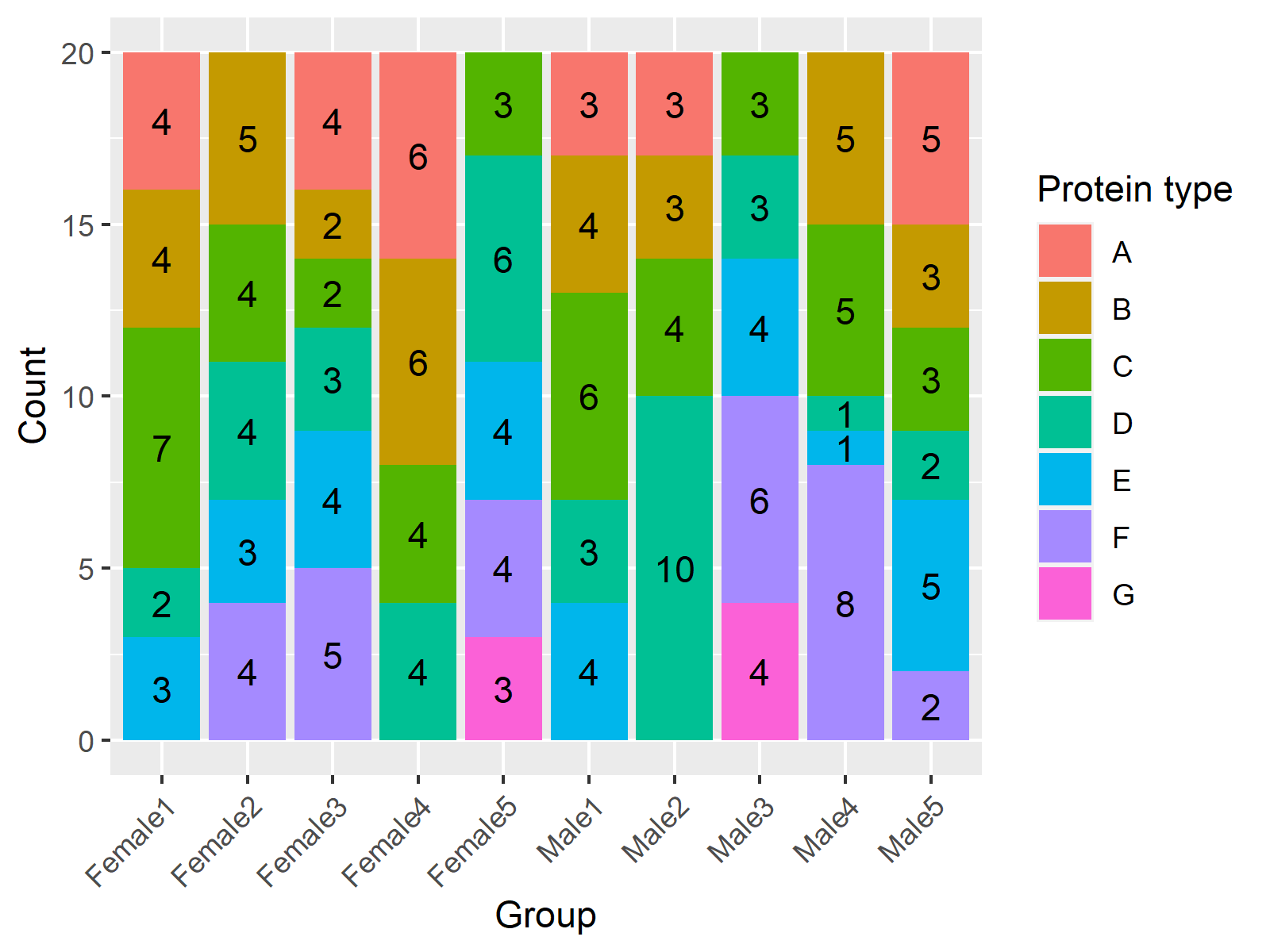I hope you find the solution helpful.

Regards,
Cansu

••May 24, 2023 7:02 am